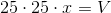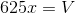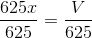## Example Questions

### Example Question #1 : Prisms

For a box to fit inside the cupboard, the sum of the height and the perimeter of the box must, at most, be 360 cm. If Jenn has a box that has a height of 40 cm and a length of 23 cm, what is the greatest possible width of the box?

207 cm

13 cm

0.4 cm

297 cm

137 cm

137 cm

Explanation:

First we write out the equation we are given. H + (2L +2W) = 360.  = 40 and = 23

40 + (2(23) + 2W) = 360

40 + (46 + 2W) = 360

46 + 2W = 320

2W = 274

W = 137

### Example Question #2 : Prisms

The volume of a rectangular prism is 80 cm3.  The length, width, and height of the prism are each an integer number of cm.  If the dimensions form three terms of an arithmetic sequence, find the average of the three dimensions.

5

8

6

4

7

5

Explanation:

Method 1:

Trial and error to find a combination of factors of 80 that differ by the same amount will eventually yield 2, 5, 8.  The average is 5.

Method 2:

Three terms of an arithmetic sequence can be written as x, x+d, and x+2d. Multiply these together using the distributive property to find the volume and the following equation results:

x3 + 3dx2 + 2d2x - 80 = 0

Find an integer value of x that creates an integer solution for d.  Try x=1 and we see the equation 1 + 3d + 2d2 - 80 = 0 or 2d2 + 3d -79 = 0.  The determinant of this quadratic is 641, which is not a perfect square.  Therefore, d is not an integer when x=1.

Try x=2 and we see the equation 8 + 12d + 4d2 - 80 = 0 or d2 + 3d - 18 = 0.  This is easily factored to (d+6)(d-3)=0 so d=-6 or d=3.  Since a negative value of d will result in negative dimensions of the prism, d must equal 3.  Therefore, when substituting x=2 and d=3, the dimensions x, x+d, and x+2d become 2, 5, and 8.  The average is 5.

### Example Question #3 : Prisms

A right rectangular prism has a volume of 64 cubic units. Its dimensions are such that the second dimension is twice the length of the first, and the third is one-fourth the dimension of the second. What are its exact dimensions?

3 x 6 x 12

4 x 4 x 4

1 x 2 x 32

1 x 4 x 16

2 x 4 x 8

2 x 4 x 8

Explanation:

Based on our prompt, we can say that the prism has dimensions that can be represented as:

Dim1: x

Dim2: 2 * Dim1 = 2x

Dim3: (1/4) * Dim2 = (1/4) * 2x = (1/2) * x

More directly stated, therefore, our dimensions are: x, 2x, and 0.5x. Therefore, the volume is x * 2x * 0.5x = 64, which simplifies to x3 = 64. Solving for x, we find x = 4. Therefore, our dimensions are:

x = 4

2x = 8

0.5x = 2

Or: 2 x 4 x 8

### Example Question #4 : Prisms

A right rectangular prism has a volume of 120 cubic units. Its dimensions are such that the second dimension is three times the length of the first, and the third dimension is five times the dimension of the first. What are its exact dimensions?

1 x 5 x 24

2 x 6 x 10

4 x 12 x 20

2 x 5 x 12

2 x 6 x 10

Explanation:

Based on our prompt, we can say that the prism has dimensions that can be represented as:

Dim1: x

Dim2: 3 * Dim1 = 3x

Dim3: 5 * Dim1 = 5x

More directly stated, therefore, our dimensions are: x, 3x, and 5x. Therefore, the volume is x * 3x * 5x = 120, which simplifies to 15x3 = 120 or x3 = 8. Solving for x, we find x = 2. Therefore, our dimensions are:

x = 2

3x = 6

5x = 10

Or: 2 x 6 x 10

### Example Question #5 : Prisms

The length of a crate is three-fourths its height and two-thirds its width. The surface area of the crate is 12 square meters. To the nearest centimeter, give the length of the box.

The correct answer is not among the other responses.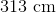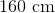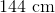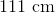Explanation:

Call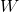, and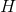the length, height, and width of the crate.

The length of the crate is two-thirds its width, so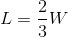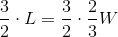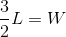The length of the crate is three-fourths its height, so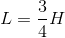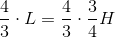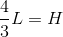The dimensions of the crate in terms ofare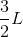, and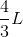. The surface area is found using the formula: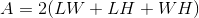Substitute: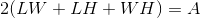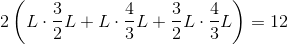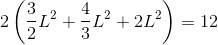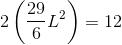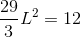Solve for: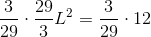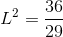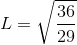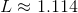meters.

Since one meter comprises 100 centimeters, multiply by 100 to convert to centimeters: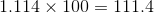This rounds to 111 centimeters.

### Example Question #6 : Prisms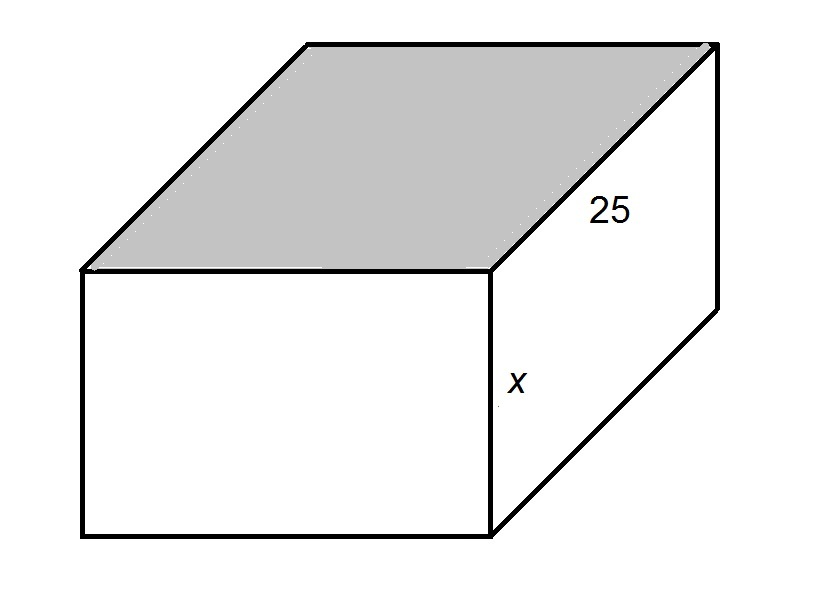The shaded face of the above rectangular prism is a square. If the volume of the prism is, givein terms of.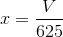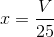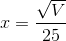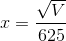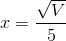Explanation:

Since the top face of the prism is a square, the common sidelength - and the missing dimension - is 25.

The volume of a rectangular prism is equal to the product of its length, width, and height - that is,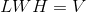Setting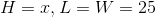, and solving for: